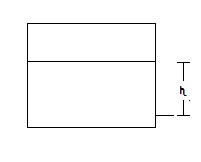INTRODUCTION

The program simulator compute the plant into two big part.

First, all the units, except the pipes, are compute, the target of the compute is to obtain the pressure in each point of the units. For example :When h is the hight of the tank.           ρ is the density of th mixture =>     P = ρgh

Second, all the pipes are compute, the target of the compute is to obtain the flowrate, in other way, the quantity of the compounds that are been moved. It allowed to know way of the compound. There is possible you have parts of the same pipe with different compounds, only 10 zone will be considerate.

BACK